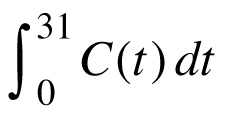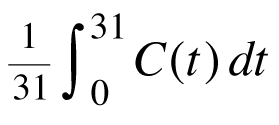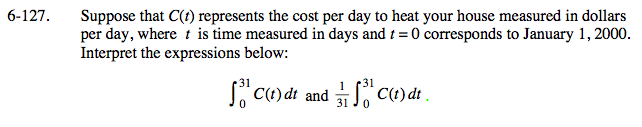### Home > CALC > Chapter 6 > Lesson 6.4.1 > Problem6-127

6-127.

Suppose that C(t) represents the cost per day to heat your house measured in dollars per day, where t is time measured in days and t = 0 corresponds to January 1, 2000. Interpret the expressions below:and. Homework Help ✎The second expression divides the first integral by 31. What does 31 represent? Why divide?
What practical information will it calculate about the cost of heat?

The first integral represents the area under the C(t) curve between t = 0 and t = 31.
Since C(t) represents cost per day, its a rate function.
The area under this rate function represents the accumulated cost during the 31 days of January.